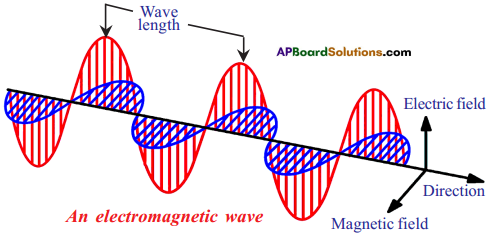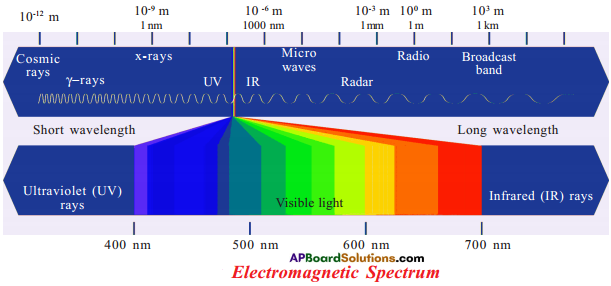# AP SSC 10th Class Chemistry Important Questions Chapter 8 Structure of Atom

AP State Board Syllabus AP SSC 10th Class Physical Science Important Questions Chapter 8 Structure of Atom.

## AP State Syllabus SSC 10th Class Chemistry Important Questions 8th Structure of Atom

### 10th Class Chemistry 8th Lesson Structure of Atom 1 Mark Important Questions and Answers

Question 1.
Write the electronic configuration of chromium. (AP June 2016)
Answrr:
The electronic configuration of chromium is
1s²2s²2p63s²3p64s¹3d5 or [Ar] 4s¹3d5

Question 2.
Out of 3d and 4s, which has more (n + l) value? Explain. (AP June 2017)
1) 3d ⇒ n + l ⇒ 3 + 2 ⇒ 5 (energy)
4s ⇒ n + l ⇒ 4 + 0 ⇒ 4 (energy)

2) Hence, ‘3d’ has more (n + l) value than ‘4s’.Question 3.
Prepare a question on nlx method. (AP SA-I:2018-19)

• How is nlx method useful.
• Explain the nlx method with an example.

Question 4.
Which colours do you observe when an iron rod is gradually heated to higher tem-peratures? (TS June 2015)
First iron turns into red (lower energy corresponding to higher wavelength) and as the temperature rises it glows and turns into orange, yellow, blue or even white respectively (higher energy and lower wavelength).

Question 5.
Which principle is not followed in writing the electronic configuration of 1s² 2s¹ 2p4? Give reasons. (TS June 2015)
1) Principle :
Aufbau principle is not followed in writing the electronic configuration of 1s² 2s¹ 2p4.

2) Reasons :
i) According to Aufbau principle electron enters into orbital of lowest energy.
ii) Between 2s and 2p, 2s has least energy. So 2s must be filled before the electron has to enter 2p.Question 6.
Write the symbol of the outermost shell of magnesium (Z = 12) atom. How many electrons are present in the outermost shell of magnesium? (TS June 2017)
Symbol of the outermost shell of magnesium (3rd shell) = M
No. of electrons in outermost shell of Magnesium = 2.

Question 7.
The four quantum number values of the 21st electrons of scandium (Sc) are given in the following table. (TS March 2017)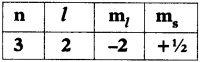Write the values of the four quantum numbers for the 20th electron of scandium (Sc) in the form of the table.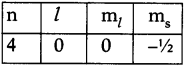Question 8.
If n = 3, mention the orbitals present in the shell and write maximum number of electrons in the shell. (TS March 2018)
(OR)
Write the maximum number of electrons and number of orbitals in the shell, when n = 3.

1. When n = 3, number of subshells = 3 (3s1 3p1 3d)
2. Number of orbitals = 9 (3s(1) 3p(3) 3d(5))
3. Maximum number of electrons (3s² 3p6 3d10) = 18

Question 9.
What is dispersion?
The splitting of light into different colours is called dispersion.

Question 10.
What is an electromagnetic wave?
When electric field and magnetic fields are perpendicular to each other and at right angles to the direction of propagation of wave is formed. Such a wave is called electromagnetic wave.Question 11.
What is a Zeeman effect?
The splitting of spectral lines in the presence of magnetic field is called Zeeman effect.

Question 12.
What is a spectrum?
Group of wavelengths is called spectrum (or) A collection of dispersed light giving its wavelength composition is called a spectrum.

Question 13.
What is speed of electromagnetic wave?
It is equal to speed of light, i.e. 3 x 108 ms-1

Question 14.
Which colour has highest wavelength and which colour has least wavelength on visible spectrum?
The colour that has highest wavelength in visible spectrum is red and least wavelength is violet.Question 15.
If n = 5, then what is the maximum value for l?
The maximum value for l is 4.

Question 16.
If l = 4, what is the number erf values for ml?
ml = 2l + 1 = 2(4) +1=9.

Question 17.
What are the values of ms?
½ or – ½

Question 18.
What is electronic configuration?
Distribution of electrons in shells, sub-shells and orbital in an atom is known as electronic configuration.

Question 19.
What is Heisenberg’s principle of uncertainty?
It is not possible to find the exact position and velocity of electron simultaneously.

Question 20.
Give ascending order of various atomic orbitals according to Moeller diagram.
1s < 2s < 2p < 3s < 3p < 4s < 3d < 4p < 5s < 4d < 5p < 6s < 4d < 5d < 6p < 7s < 5f < 6d < 7p < 8s.

Question 21.
What is Hund’s Rule?
Electron pairing in orbitals starts only when all available empty orbitals of the same energy are singly occupied.Question 22.
What is Planck’s equation?
Planck’s equation is E = hv.
E = Energy of the radiation
h = Planck’s constant = 6.625 × 10-34 J

Question 23.
What is electromagnetic spectrum?
Electromagnetic waves can have a wide variety of wavelengths. The entire range of wavelengths is known as the electromagnetic spectrum.

Question 24.
What is wavelength?
The distance from one wave peak to the next is called wavelength (λ).

Question 25.
What is frequency?
The number of wave peaks that pass by a given point per unit time is called frequency.Question 26.
When are electromagnetic waves produced?
AnElectromagnetic waves are produced when an electric charge vibrates.

Question 27.
Which is the example for line spectrum?
The atomic spectrum of hydrogen atom.

Question 28.
Which model explains fine spectrum of atom?
Bohr – Sommerfeld model.

Question 29.
How are wavelength and velocity of light related?
c = vλ
where
c = velocity of light,
v = frequency of light,
λ = wavelength of light.

Question 30.
Give the equation which gives electromagnetic energy (light) that can have only Certain discrete energy values.
E = hv
E = Energy of light
h = Planck’s constant = 6.62 5 × 10-27 erg sec or 6.625 × 10-34 Joule-sec
This equation is called Planck’s equation.Question 31.
Which group elements are called Noble gases?
VIII A group or 18th group elements are called inert gases (or) Noble gases.

Question 32.
Which elements are highly stable?
Noble gases are highly stable.

Question 33.
Write the set of quantum numbers for the electrons in a 3pz orbital.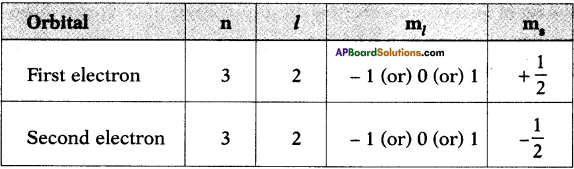Question 34.
What is the difference between an orbit and orbital?
An orbit is a well defined path of electron that revolves around the nucleus.

An orbital is the space around the nucleus, where the probability of finding electrons is maximum.

Question 35.
What are the factors which influence electromagnetic energy?
Electromagnetic energy depends on two factors

1. wavelength
2. frequency.

Question 36.
What is a wave?
The disturbance occurred in a medium is called wave.Question 37.
When cupric chloride is kept in non-luminous flame then what is the colour of flame?
Green colour.

Question 38.
If the colours gradually changes there are no sharp boundaries in between them, then what is the name given to that type of spectrum?
Continuous spectrum of emission.

Question 39.
What is the information given by magnetic orbital quantum numbers?
Orientation of orbitals in space.

Question 40.
How many orbitals are present in a sub-shell?
The number of orbitals are present in a sub-shell is n² (where n is principal quantum number).

Question 41.
What happens when an object is suitably excited by heating?
Light is emitted by the object.

Question 42.
What is meant by Aufbau?
The German word Aufbau means building up.

Question 43.
Which elements are examples for Noble gases?
Helium (He), Neon (Ne), Argon (Ar), Krypton (Kr), Xenon (Xe) and Radon (Rn) are examples for Noble gases.

Question 44.
Which element has duplet configuration?
Helium (1s²)Question 45.
Write the set of quantum number for the added electron of oxygen atom.
Configuration of oxygen is 1s² 2s² 2p4.
The added electron is 4th in the 2p.
The set of quantum numbers (2, 1, -1, -½)

Question 46.
Can we apply c = vλ, to sound waves?
Yes. It is a universal relationship and applies to all waves.

Question 47.
What is the value of Planck’s constant?
The value of Planck’s constant is 6.626 × 10-34 Js.

Question 48.
Why do different elements emit different flame colours when heated by the same non-luminous flame?
The light emitted by different kind of atoms is different because the excited states electrons will go are different.

Question 49.
How many values can ‘l’ have for n = 4?
If n = 4, l can take values 0, 1, 2, 3. So there are four values.

Question 50.
Write the four quantum numbers for the differentiating electrons of lithium (Li) atom.
The electronic configuration of lithium is 1s² 2s¹. So differentiating electron enters into 2s. The values of four quantum numbers are as given below.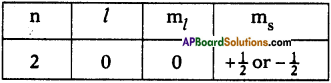Question 51.
Write four quantum numbers for 2p¹ electrons.
The four quantum numbers for 2p are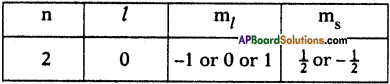Question 52.
Which rule is violated in the following electronic configuration?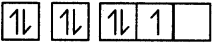The rule violated is Hund’s rule.

Question 53.
How many maximum number of electrons that can be accommodated in N principle energy shell?
For N shell n = 4.
The maximum number of electrons accommodated in a shell is 2n².
∴ A maximum of 32 electrons can be filled in N shell.Question 54.
How many maximum number of electrons that can be accommodated in a ‘l’ sub-shell?
l sub-shell has 3 orbitals. Each orbital accommodates 2 electrons. So 6 electrons can be filled in l sub-shell.

Question 55.
How many maximum number of electrons can be accommodated in ‘d’ sub-shell?
d sub-shell has 5 orbitals. So 10 electrons can be filled in d sub-shell.

Question 56.
How many sub-shells present in a ‘M’ principal energy shell?
For M shell n = 3
The number of sub-shells in M shell is 3.

Question 57.
How many spin orientations are possible for an electron in s-orbital?
The spin quantum number values for electrons are ½ or -½. So 2 spin orientations are possible.

Question 58.
Write valence electronic configuration of element which has the following set of quantum numbers.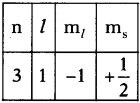n = 3 indicates 3rd orbit and l = 1 indicates p orbital and there is one electron in p orbital. So the valence electron configuration is 3p¹.

Question 59.
How many unpaired electrons are present in chromium?
The electronic configuration of chromium 1s² 2s² 2p6 3s² 3p6 4s¹ 3d5.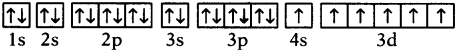The number of unpaired electrons = 6.

Question 60.
Find the four quantum number values of 3rd and 4th electrons of Beryllium.
The electronic configuration of Beryllium is 1s² 2s².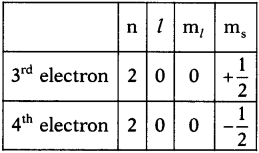Question 61.
What is the n + l value of 4f orbital?
For 4f orbital n = 4 and f orbital l = 3.
∴ n + l = 4 + 3 = 7

Question 62.
When you heat iron rod first it turns red. Why?
Iron turns into red because red has higher wavelength. So it has lower energy which is emitted by iron.Question 63.
What is the significance of Planck’s proposal?
Electromagnetic energy can be gained or lost in discrete values and not in a continuous manner.

Question 64.
When do you see an emission line?
The energy emitted by an electron is seen in the form of an electromagnetic energy and when the wavelength is. in the visible region it is visible as an emission line.

Question 65.
How many elliptical orbits are there in 4th orbit of Sommerfeld?
The number of elliptical orbits in 4th orbit of Sommerfeld is 3.

Question 66.
Why is spin quantum number introduced?
When we observe spectrum of yellow light by using high resolution spectroscope it has very closely spaced doublet. Similar patterns are shown by Alkali and Alkaline earth metals. In order to account this spin quantum number is introduced.

Question 67.
Which of the following magnetic quantum number values is not possible for 3d orbital?
a) – 2
b) – 1
c) 0
d) 4
For d orbital the possible m; values – 2, – 1, 0, 1, 2. So the value 4 is not possible.

Question 68.
If an element has 11 electrons in its M shell, then what is the name of element and its atomic number?
The electronic configuration of element is 1s² 2s² 2p6 3s² 3p6 4s² 3d3.
[M shell electrons = 2 + 6 + 3=11]

So the element is Titanium.

Question 69.
The wave length of a wave is 100 nm. Find its frequency.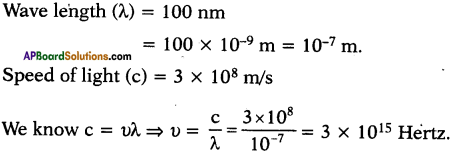### 10th Class Chemistry 8th Lesson Structure of Atom 2 Marks Important Questions and Answers

Question 1.
Explain Hund’s Rule with an example. (TS March 2016) (AP SA-1:2018-19)
Hund’s Rule :
According to this rule electron pairing in orbitals starts only when all available empty orbitals of the same energy are singly occupied.

(1) Ex :

1. The configuration of carbon atom (Z = 6) is 1s² 2s² 2p².
2. The first four electrons go into the 1s and 2s orbitals.
3. The next two electrons go into 2px and 2py orbitals.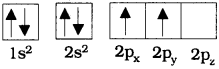4. But, they do not pair in 2px orbital.

(2) Ex :

1. In oxygen atom (8O), distribution of electrons is given below.
2.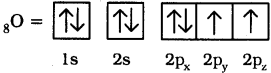3. Here, pairing of electrons in 2px orbital takes place after, 2px, 2py and 2pz orbitals are filled with a single electron.

Question 2.
The electronic configuration of Sodium is 1s² 2s² 2p6 3s¹. (AP March 2017)
What information that it gives?

1. Its atomic number is 11
2. It is s-block element
3. It is in 3rd period ’
4. It is in 1st group
5. Its valency is 1
6. Number of valency electrons are 1
7. It can form uni positive ion
8. It can form ionic bond, 9. It is metal.

Question 3.
Explain the principle which describes the arrangement of electrons in degenerate orbitals.
According to Hund’s rule the degenerate orbitals are occupied with one electron each before pairing of electron starts.
Ex : Electronic configuration of carbon is 1s² 2s² 2p²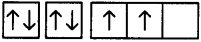the last two electrons will enter into separate 2p orbitals.

Question 4.
Name the principle, which says an orbital can hold only 2 electrons and explain. (AP March 2018)

• Name of the principle : Pauli’s exclusion principle.
• No, two electrons in an orbital can have all four quantum numbers same.
• It says there is a chance to hold only 2 electrons, one rotates in clockwise direction (+½)other rotates in anticlock wise direction (-½).Question 5.
For a better understanding about the electronic configuration in an atom, the teacher wrote shorthand notation nlx on the blackboard.
Looking at this notation, what could be the probable questions that generate in the student’s mind? Write any two of them. (TS March 2015)

1. What n, l, x indicates related to atoms?
2. How nlx indicates the position of the electrons in the atom?

Question 6.
Write the ‘Octet Rule’. How does Mg (12) get stability while reacting with chlorine as per this rule? (TS June 2017)
Octet Rule :
The atoms of elements tend to undergo chemical changes that help to leave their atoms with eight outer – shell electrons.

Magnesium atom looses 2 electrons and get 8 electrons in its outermost shell as Neon. So that it gets stability.

Question 7.
Write the electronic configuration of the atom of an element having atomic number 11. Write the names of the rules and the laws followed by you in writing this electronic configuration. (TS March 2017)
1s²2s²2p63s¹.
(OR)
Principles followed :

1. Aufbau principle.
2. Hund’s Rule.
3. Pauli Exclusion Principle

Question 8.
The electron enters into 4s orbital after filling 3p orbital but not into 3d. Explain the reason (TS March 2018)
Based on (n+l) values energy value of 3d orbitals is 3 + 2 = 5, energy value of 4s orbitals is 4 + 0 = 4
The energy level of 4s orbital is less than the 3d orbital according to the Aufbau principle electron enters into lower energy orbital first.
Thats why electrons enters into 4s orbital after filling 3p, but not into 3d.Question 9.
Write the electronic configuration of Na+ and Cl.
Electronic Configuration of Na+ is 1s² 2s² 2p6 and Cl is 1s² 2s² 2p6 3s² 3p6.

Question 10.
Observe the given table and answer the following questions. (TS March 2019)

 Sl.No. Electron Configuration 1. 1s2 2s2 2p6 3s2 3p3 2. 1s2 2s2 2p6 3s2 3p6 4s2 3. 1s2 2s2 2p6 3s2 3p6

1) Mention the divalent element name.
2) Name the element belongs to 3rd period and VA Group.

1. Name of the divalent element in the table is Calcium.
2. Name of the element which belongs to 3rd period and VA Group is Phosphorous.

Question 11.
Your friend is unable to understand nlx. What questions will you ask him to understand nlx method? (AP SCERT: 2019-20)

1. What is nlx method?
2. Where does it use ?
3. What is meant by ‘n’, 7′ and ‘x’?
4. How can we use nlx method in the writing of electronic configuration?

Question 12.
Why do valency electrons involve in bond formation, than electrons of inner shells? (AP SCERT: 2019-20)

1. When two atoms come sufficiently close together the valence electrons of each atom experience the attractive force of the nucleus in the other atom.
2. The nucleus and the electrons in the inner shell remain unaffected when atoms come close together.
3. The electrons in outer most shell of an atom get affected.
4. Thus electrons in valence shell are responsible for the formation of bond between atoms.

Question 13.
Explain Pauli’s exclusion principle with an example. (AP SA-I:2019-20)
Pauli’s exclusion principle :
No two electrons of the same atom can have all four quantum numbers the same. If n, l and ml are same for two electrons, then ms must be different.
Suppose take the example of Helium atom.
The four quantum numbers for two electrons in the Helium atom given below.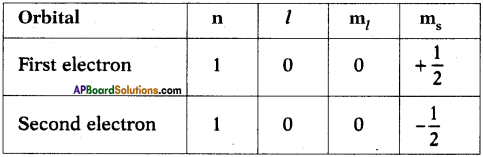We can observe from the table that three of the quantum numbers are same but fourth quantum number is different. The electronic configuration of Helium atom is² ↑↓. So the maximum number of electrons filled in an orbital is 2.

Question 14.
Explain Aufbau principle. (AP SA-I:2019-20)
Aufbau principle :

1. In the ground state the electronic configuration can be built up by placing electrons in the lowest available orbitals until the total number of electrons added is equal to atomic number.
2. Thus orbitals are filled in the order of increasing energy.
3. Electrons are assigned to orbitals in order of increasing value of n + l.
4. For sub-shells with the same value of n + l, electrons are assigned first to the subshell with lower n.Question 15.
The electronic configuration of an atom is as follows 1s² 2s² 2p².
a) Which element’s atom is it?
b) Which orbital is the last electron in?
c) When excited what could be the number of lone / single electrons in this atom?
d) What is the value of principal quantum numbers of two electrons in the first box?
Given electronic configuration of atom is 1s² 2s² 2p².
a) The element is carbon.
b) The valence electron enters 2p orbital.
c) In excited state the electron in 2s orbital enters 2p orbital. So it has 4 unpaired electrons.
d) The value of principal quantum number is 1.

Question 16.
Draw the table which gives the information about the quantum numbers and the number of the quantum states.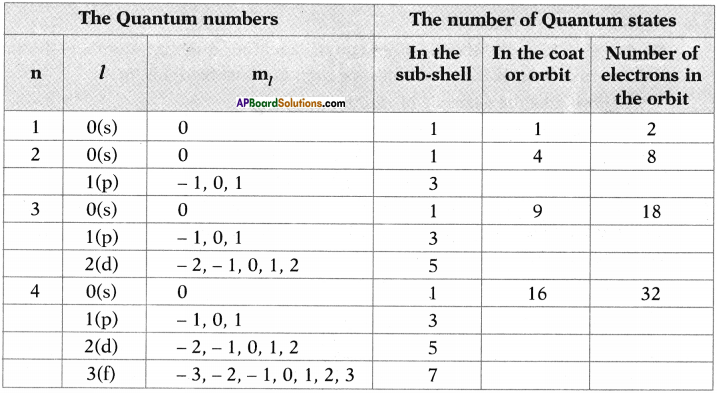Question 17.
Explain briefly about spin quantum number.

• This gives spin of the electrons about their own axes. It is denoted by ms.
• This quantum number refers to the two possible orientations of the spin of an electron, one clockwise and the other anti-clockwise spin.
• These are represented by +½ -½ and .

Question 18.
Write electronic configurations of following elements,
a) Hydrogen
b) Helium
c) Lithium
d) Beryllium
e) Boron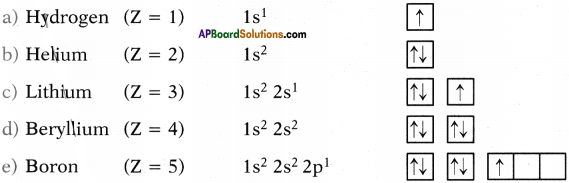Question 19.
What does a line spectrum tell us about the structure of an atom?
The electrons in ground state i.e. lowest, energy state absorb energy and move into excited state where they are unable to stay for long periods so lose the energy and come back to the ground state. The emitted radiation appears as line in line spectrum.

Question 20.
What are the spins of electrons in Helium atom?
The quantum numbers for two electrons of Helium are given below as per Pauli’s exclusive principle.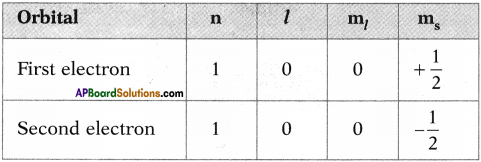Three of quantum numbers are same. So fourth must be different so the two electrons have anti-parallel spins.

Question 21.
1s² 2s² 2p6 3s² 3p6 3d10 4s¹ is the electronic configuration of Cu (Z = 29). Which rule is violated while writing this configuration? What might be the reason for writing this configuration?
The rule violated is Aufbau principle. The elements which have half filled or completely filled orbitals have greater stability. So copper can get stability by transferring one electron from 4s to 3d (their energies are close to each other).

So the electronic configuration of copper is 1s² 2s² 2p6 3s² 3p6 3d10 4s¹, not 1s² 2s² 2p 3s² 3p6 3d9 4s².

Question 22.
Why are chromium and copper exceptions to electronic configuration?
Elements which have half-filled or completely filled orbitals have greater stability. So in chromium and copper the electrons in 4s and 3d redistribute their energies to attain stability by acquiring half-filled and completely filled d orbitals.

Hence the actual electronic configurations of chromium and copper are as follows.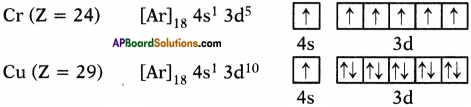Question 23.
Distinguish between emission and absorption spectrum.

 Emission spectrum Absorption spectrum 1) The spectrum produced by emitted radiation is called emission spectrum. 1) The spectrum produced by absorption of radiation is called absorption spectrum. 2) The emission spectrum contains bright lines on the dark back-ground. 2) The absorption spectrum contains dark lines on the bright back-ground. 3) The emission spectrum corresponds the radiation emitted when an excited electron comes back to the ground state. 3) The absorption spectrum corresponds the radiation absorbed in exciting an electron from lower to higher energy levels.

Question 24.
Draw a table which will give the relationship between l values and number of orbitals and name of sub-shell and maximum number of electrons.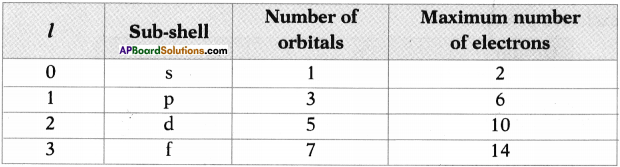Question 25.
Distinguish between line and band spectrum.

 Line spectrum Band spectrum 1) The spectrum has sharp distinct lines. 1) The spectrum has many closed, spaced lines. 2) The spectrum is characteristic of atoms and is also called atomic spectrum. 2) The spectrum is characteristic of molecules is also called molecular spectrum. 3) The spectrum is given by inert gases, metal vapours and atomised non-metals. 3) The spectrum is given by hot metals and molecular non-metals.

Question 26.
What is Dispersion of light? Explain a natural example of dispersion of light.
Dispersion:
Splitting of white light into colours (VIBGYOR) is called Dispersion of light.

The natural example for dispersion of light is formation of Rainbow. It is caused by dispersion of sunlight by tiny water droplets present in atmosphere which act as small prisms.Question 27.
Wien is an electromagnetic wave produced? Write about characteristics of electromagnetic wave.
Electromagnetic wave is produced when an electric charge vibrates (moves back and fortn).

Characteristics of electromagnetic waves :

1. Efectric field and magnetic fields are perpendicular to each other and at right angles to direction of propagation of wave.
2. It travels with speed of light i.e., 3 × 108 ms-1.
3. Electromagnetic energy is characterized by wavelength (λ) and frequency (v). The relation is given by c = vλ.

Question 28.
The valence electron configuration of element is given as 4s¹. Then give the following information.
1) What is the name of that element?
2) What is the outermost orbit of element?
3) What is ‘l’ value of outermost sub-shell?
4) What is the atomic number of element?

1. Potassium.
2. N
3. The outermost sub-shell is 4s its l value is ‘O’.
4. Its electronic configuration is 1s² 2s² 2p6 3s² 3p6 4s¹. So its atomic number is 19.

Question 29.
Write all the quantum numbers for valance electron of sodium.

1. The electronic configuration of sodium is 1s² 2s² 2p6 3s¹.
2. The valance orbital is 3s.
3. The quantum numbers for this orbital is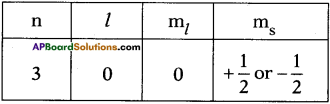Question 30.
Give electronic configurations of following elements.
a) Sodium
b) Phosphorous
Sodium – 1s² 2s² 2p6 3s¹
Phosphorous – 1s² 2s² 2p6 3s² 3p³

Question 31.
Why does nitrogen has more chemical stability when compared with oxygen?
The electronic configurations of Nitrogen and Oxygen are as follows.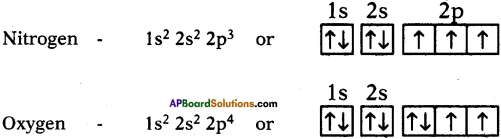Nitrogen has half filled 2p³ configuration. So it has greater chemical stability when compared with Oxygen.

Question 32.
Ramu gave electronic configuration of potassium as 1s² 2s² 2p6 3s² 3p6 3d¹ whereas Ravi expressed the configuration as 1s² 2s² 2p6 3s² 3p6 4s¹. Who gave the correct configuration? Why?
Ravi gave the correct configuration because according to Aufbau principle after completion of 3p orbital electron may enter either 4s or 3d.
Their n + l values are given below.

 Orbital n + l Values 4s 4 + 0 = 4 3d 3 + 2 = 5

So 4s orbital has lower n + l value when compared with 3d orbital. So electron enters into 4s.

Question 33.
Given the valence electron configuration of an element is 4s¹. Then what are its quantum number values. Which element does it represent?
Given valence electronic configuration is 4s¹. So n = 4 for ‘s’ sub shell l = 0 and if l is ‘O’ then ml is also zero. ms takes only two values that is +½ or -½ for convenience we can take ms as +½.
∴ The quantum number values are like this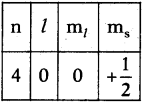The element is potassium.

Question 34.
The atomic number of an element is 17, then calculate the total number of electrons present in its s and p orbitals.
The element with atomic number 17 is chlorine.
Its electronic configuration is 1s² 2s² 2p6 3s² 3p5.
So, the total number of electrons present in s orbitals = 2 + 2 + 2 = 6.
The total number of electrons present in p orbitals = 6 + 5 = 11.Question 35.
Based on Aufbau’s principle, in which of the three 4d, 5p and 5s orbitals the electrons will be filled first? Why?
According to Aufbau’s principle, the electron enters the orbital having lower n + l value. If both orbitals have same n + l values, electron enters the orbital with lower ‘n’ value.

 Oprbital n + l value 4d 4 + 3 = 7 5p 5 + 1 = 6 5s 5 + 0 = 5

So, 3s has least n + l value. Therefore the electron enters 5s orbital first.

Question 36.
Find the following.
1) Number of orbitals present in M orbit.
Number of orbitals present in an orbit = n² For M orbit n = 3.
∴ So the number of orbitals = 3² = 9.

2) The maximum and minimum possible ml values for 4f orbital.
For f orbital ‘l’ value is 3. If l is 3, then ml takes values from – 3 to + 3.
So the minimum value for ml is – 3 and maximum value is + 3.

3) The possible values of l if n = 4.
If n = 4, then l take values from 0 to 3 i.e., 0, 1, 2, 3.

4) The maximum number of electrons that can be filled in ‘N’ energy level.
For N orbit n = 4. The maximum number of electrons present in a shell = n².
∴ The maximum number of electrons filled in N shell = 4² = 16.

Question 37.
How do the vibrating electric and magnetic fields around the charge become a wave that travel through space?

• A vibrating electric charge creates a change in the electric field.
• The changing electric field creates a changing magnetic field.
• This process continues, with both the created fields being perpendicular to each other and at right angles to the direction of propagation of the wave.Question 38.
What information do the quantum numbers provide?
The quantum numbers describe the space around the nuclear where the electrons are found and also their energies.

Question 39.
An electron in an atom has the following set of four quantum numbers. Which orbital does it belong to?

 n l ml ms 3 0 0 +½

The quantum numbers of an atom is given below.

 n l ml ms 3 0 0 +½

By using nlx method n = 3, if l = 0 then the sub-shell is s.
So the electron belongs to 3s.

Question 40.
Look at the following table and answer the following questions.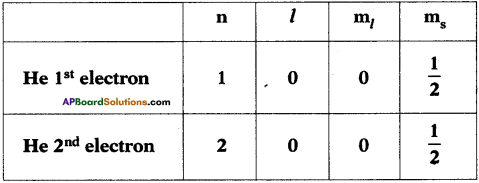a) What is the law that is voilated in the above table? State that law.
b) Write the correct table using that law.
a) The law violated is Pauli’s Exclusive Principle. Pauli’s law states that no two electrons of the same atom can have all the four quantum numbers same.
b)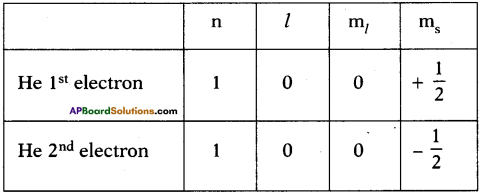### 10th Class Chemistry 8th Lesson Structure of Atom 4 Marks Important Questions and Answers

Question 1.
Draw Moeller chart of filling order of atomic orbitals.
(OR)
Draw a diagram showing the increasing value of (n + l) of orbitals. (AP June 2017)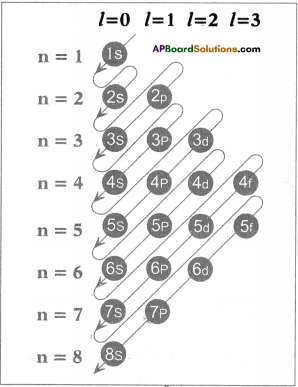The filling order of atomic orbitals (Moeller Chart)

Question 2.
Complete the following table based on quantum numbers related to atomic orbitals and electron of an atom. (AP SA-I-2018-19)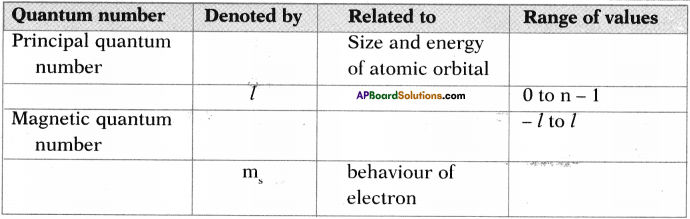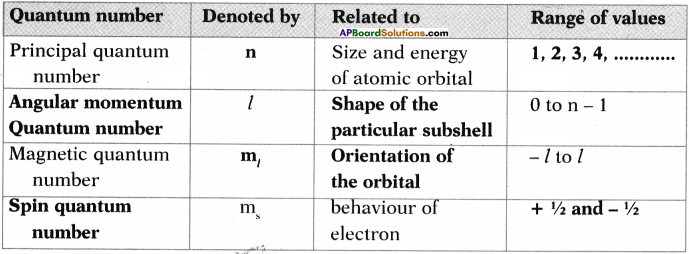Question 3.
Based on the information given in the table, answer the questions given below.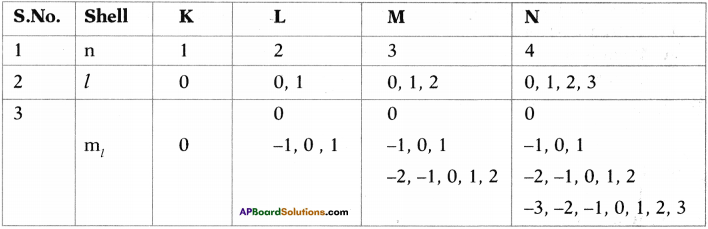i) For the 4th main shell, how many values are there for ml? What are they? (TS June 2016)
1) There are 16 m( values in the 4th main shell.
2) They are

 Orbital ml values Total 4s 0 1 4p -1, 0, + 1 3 4d -2, -1, 0, + 1, +2 5 4f -3, -2, -1, 0, +1, +2, +3 7 Total 16

ii) For sub-shell with n = 3, l = 1, write the m, values.
For sub – shell with n = 3, l = 1 the m, values are -1, 0, 1.

iii) Write the principal quantum number value for ‘N’ shell. How many sub-shells are there in the main shell?

1. The principal quantum number value for ‘N’ shell is 4.
2. The number of sub-shells is 4. They are 4s, 4p, 4d, 4f.

iv) In the above table ml and l values are given. Write a formula that gives the relationship between ml and l based on those values.
ml (No. of values) = 2l + 1.

Question 4.
Observe the information provided in the table about quantum numbers. Then answer the questions given below it. (TS June 2017)

 n l ml 1 0 0 2 0 0 1 -1, 0, +1 3 0 0 1 -1, 0, +1 2 -2, -1, 0, +1, +2

i) Write the ‘l’ value and symbol of the spherical shaped sub-shell.
ii) How many values that ‘ml‘ takes for 1 = 2? What are they?
iii) Write the symbols of the orbitals for l = 1 sub-shell.
iv) What is the shape of the sub-shell for l = 2? What is the maximum number of electrons that can occupy this sub-shell?
i) Spherical shaped sub-shell “l” value is zero and symbol is ‘s’.
ii) Number of m; values for 1 = 2 is 5, those are -2, -1, 0, 1, 2.
iii) Symbols of the orbitals for l = 1 sub-shell are px, py, pz.
iv) Shape of the subshell l = 2 is double dumbel.
The maximum number of electrons that can occupy in this sub-shell is 10.

Question 5.
Write postulates and limitations of Bohr Hydrogen atomic model. (TS June 2018)
Postulates :

1. Niels Bohr proposed that electrons in an atom occupy ‘stationary’ orbits of fixed energy at different distances from the nucleus.
2. When an electron jumps from a lower energy state (ground state) to higher energy state it absorbs energy or emits energy when such a jump occurs from a higher energy state.
3. The energies of an electron in an atom can have only certain values E1, E2, E5…. that is, the energy is quantized.

Limitations :

1. Bohr’s model failed to account for splitting of line spectra of hydrogen atom into liner lines.
2. Bohr theory could not explain the Zeeman and Stark effect.Question 6.
Explain Bohr’s model of hydrogen atom and its limitations. (March 2019)
Niels Bohr proposed that,
a) electrons in an atom occupy stationary orbits of fixed energy (K, L, M, N,…) at different distances from the nucleus.

b) when an electron jumps from a lower energy state to higher energy state, it ab¬sorbs energy or emits energy when such a jump occurs from a higher energy state to lower energy state.

c) the energies of an electron in an atom can have only certain values E1, E2, E3, ……… i.e. the energy is quantized. The states corresponding to these energies are called stationary states and the possible values of the energy are called energy levels.

d) the angular momentum of electron is multiple integral of $$\frac{L}{2 \pi}$$.
∴ L = mvr = $$\frac{\mathrm{nh}}{2 \pi}$$
m = mass of electron ;
v = velocity of electron ;
r = radius of circular path;
h = plank constant

Limitations :

1. Bohr’s model failed to account for splitting of line spectra (Zeeman effect).
2. This model failed to account for the atomic spectra of atoms of more than one electron.
3. Bohr theory was not explained the quantisation of angular momentum of an electron.
4. It was not explained the formation of chemical bonds.

Question 7.
Explain four quantum numbers with an example. (AP SA-I; 2019-20)
Each electron iii an atom is described by a set of three numbers called Quantum numbers.

1) Principal quantum number (n) :
It is used to know the size and energy of the main shell The values of ‘n’ are 1, 2, 3 …..
energy of the shell, n = 1 < energy of the Shell n = 2.

2) Angular momentum of quantum number (l) :
It is used to know the shape of a particular sub shell.
The values of ‘l’ are 0, 1, 2, 3
l = 0 = s orbital = spherical in shape
l = 1 = p orbital = dumbel in shape
l = 2 = d orbital = double dumbel Shape

3) i) Magnetic quantum number (ml) :
It is used to describe the orientation of the orbital in space relative to the other orbitals in the atom.
The values of mt are – ‘l’ to ’+l’ including zero,

ii) Spin quantum number (ms) :
It is used to know the orientation of the spins of electrons.
The values of ms are +½ and – ½.

Question 8.
How does Hund’s rule helps in writing electronic configuration of an atom? Explain with a suitable example. (TS June 2019)
Hund’s rule :
According to this rule electron pairing in orbitals starts only when all available empty orbitals of the same energy are singly occupied.

This Hund’s rule helps in writing of electronic configuration of an element.

Example :

1. The electronic configuration of carbon (C) atom (Z = 6) is 1s² 2s² 2p6.
2. The first four electrons go into the Is and 2s orbitals.
3. The next two electrons go into separate 2p orbitals, with both electrons having the same spin.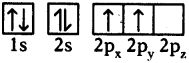Another example :

1. The electronic configuration of oxygen (8O) is 1s² 2s² 2p4.
2. The first four electrons go into the 1s, 2s orbitals.
3. The next four electrons go into 2p orbits as 2 in 2px, 1 in 2py and 1 in 2pz orbital.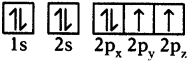4. Here pairing of electron in 2px starts after filling of electron in each 2px, 2py, 2pz orbitals.
5. But electrons do not occupy like this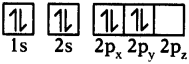Question 9.
Your father asked you to go to the market and purchase an electric lamp. The shop-keeper displayed two lamps – one is violet and another is red. Which coloured lamp do you purchase to put in your bedroom? Support your choice of solution.
Red has highest wavelength and violet has lowest wavelength. We know that relationship between energy as follows.
E = hv = h$$\frac{c}{\lambda}$$

h and c are remains constant.
∴ E ∝ $$\frac{1}{\lambda}$$

Energy is inversely proportional to wavelength. Red coloured light has lower energy and least intensity. So red coloured lamp is preferable as bed light.Question 10.

• According to Bohr, electrons revolve around nucleus in definite paths or orbits. So the exact position of the electron at various times will be known to us.
• In order to explain Bohr’s postulate we have to know the velocity and exact position of electron.
• In order to find the position of electron we have to take the help of suitable light to find the position. As the electrons are very small, light of very short wavelength is required for this task.
• This short wavelength light interacts with the electron and disturbs the motion of the electron.
• Hence, it is not possible to find the exact position and velocity of electron simultaneously. This was stated by Heisenberg which is called Heisenberg’s principle of uncertainty.
• In this way Heisenberg contradicts Neils Bohr.

Question 11.
Explain Bohr-Sommerfeld model of an atom. What is the merit of this model? What are its limitations?

• In an attempt to account for the structure of line spectra, Sommerfeld modified Bohr’s atomic model by adding elliptical orbits.
• While retaining the first of Bohr’s circular orbit as such, he added one elliptical orbit to Bohr’s second orbit, two elliptical orbits to Bohr’s third orbit, etc.
• Nucleus of the atom is one of the principal foci of these elliptical orbits because periodic motion under the influence of a central force will lead to elliptical orbits with the force situated at one of the foci.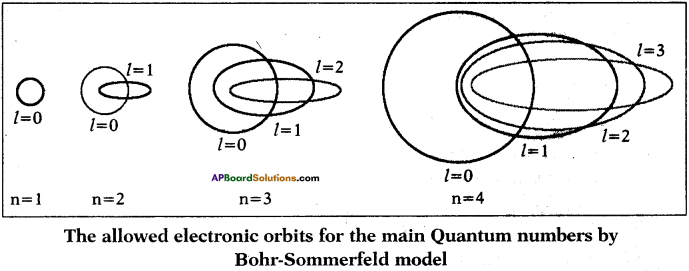Merit:
Bohr-Sommerfeld model is successful in accounting for the fine line structure of hydrogen atomic spectra.

Limitations :

1. This model failed to account for the atomic spectra of atoms of more than one electron.
2. It did not explain Zeeman and Stark effects.

Question 12.
In an atom the number of electrons in N-shell is equal to the number of electrons in K, L and M shells. Answer the following questions.
i) Which is the outermost shell?
The outermost shell is p(n = 6).

ii) How many electrons are there in its outermost shell?
Two electrons are there in outermost shell.

iii) What is the atomic number?
Its atomic number is 56.

iv) Write the electronic configuration of the elements.
The electronic configuration of element is
1s² 2s² 2p6 3s² 3p6 4s² 3d10 4p6 5s² 4d10 5p6 6s².Question 13.
Explain the following electron configurations by using nlx method.
a) 2p¹
b) 3d5
c) 4f9
d) 6s²
In nlx method n is the principle quantum number and l is the angular momentum quantum number and x is number of electrons. Now let us explain the following configurations
a) 2p¹ – It indicates that there is one electron in ‘p’ sub-shell of second orbit or shell.
b) 3d5 – It indicates that there are five electrons in ‘d’ sub-shell of third orbit or shell.
c) 4f9 – It indicates that there are nine electrons in ‘f’ sub-shell of fourth orbit or shell.
d) 6s² – It indicates that there are two electrons in ‘s’ sub-shell of sixth orbit or shell.

Question 14.
In an atom the number of electrons in L shell is equal to three times of K shell. Then answer the following.
1) Which is the outermost shell?
2) How many electrons are there in outermost shell?
3) What is the atomic number of element?
4) Write electronic configuration of element.
5) Write name of element.
Given that the number of electrons in L shell is three times of K shell.
We know that number of electrons in K shell is 2.
Therefore number of electrons in L shell = 3 × 2 = 6

1. So the outermost shell is L.
2. The number of electrons in outermost shell is 6.
3. The atomic number of element is 8.
4. The electronic configuration of element is 1s² 2s² 2p4.
5. The element is oxygen.

Question 15.
We know that the electron configuration of copper is [Ar] 4s¹ 3d10. Is it against to Aufbau principle or not. If so, why is the configuration violated?

• The atomic number of copper is 29. So its electron configuration should be [Ar] 4s² 3d4.
• But if one electron from 4s orbital jumps into 3d orbital, then copper gets half filled ‘d’ orbitals which gives stability to the atom.
• The energy difference between 4s and 3d is very less. So one electron can easily jump from 4s to 3d which gives half-filled 3d5 configuration.
• So, in order to get additional stability, Aufbau principle is violated i.e., electron enters the orbital of higher energy before the completion ortrital of lower energy.

Question 16.
Electronic configurations of following elements are written wrongly. Correct those configurations with proper explanation.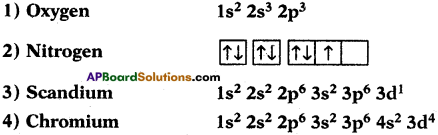1) The electron configuration of oxygen is 1s² 2s² 2p4 because the maximum number of electrons that can be filled in s orbital is 2 and so the extra electron should be entered in 2p.

2) The correct electron configuration of nitrogen is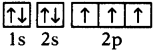The reason is that the pairing of electrons does not take place until each degenerate orbital is filled with one electron each (Hund’s principle).

3) The correct electronic configuration of scandium is 1s² 2s² 2p6 3s² 3p6 4s¹ because after completion of 3p orbital electron enters in 4s because the energy of 4s orbital is less than 3d (Aufbau principle).

4) The correct electronic configuration of chromium is 1s² 2s² 2p6 3s² 3p6 4s¹ 3d5. Because atoms having half filled or completely filled orbitals are more stable. So by transferring one electron from 4s to 3d the atom gets extra stability.

Question 17.
Here is set of quantum numbers. Do they form correct values of quantum numbers or not. If not, give reason.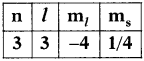The values l, ml, ms are not correct values for given ‘n’.

• The maximum value for l is n – 1. If n = 3, then l takes values from 0 to 2. That is 0, 1, 2.
• ml values depend on ‘l’. ml take values from – l to + l including zero. So, the possible values for m7 may be from – 2 to + 2.
• Spin quantum number has only two values, i.e. and –$$\frac{1}{2}$$ and $$\frac{1}{2}$$. So $$\frac{1}{4}$$ value is not possible.Question 18.
a) If n = 4, then what energy level does it represent?
If n = 4, then it represents N energy level.

b) If n = 5, then what is the maximum value for l and why?
If n = 5, then the maximum value of l for 4 because the maximum value for l is n- 1.

c) If l = 3, then what are the maximum possible values for ml?
Given l = 3.
Then possible values for ml is 2l + 1.
∴ Maximum possible values = 2(3) +1 = 7

d) What is the number of electrons present in M energy level?
For M energy level n = 3.
The maximum number of electrons in an orbit = 2n² = 2 × 3² = 2 × 9 = 18

Question 19.
Draw the shapes of s, p and d orbitals.
s – orbital (Spherical) :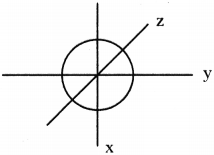p – orbital (Dumbell) :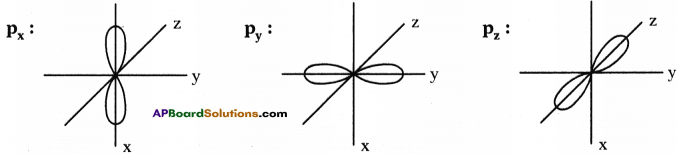All P: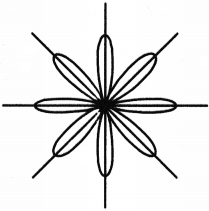d – orbital (Double Dumbell):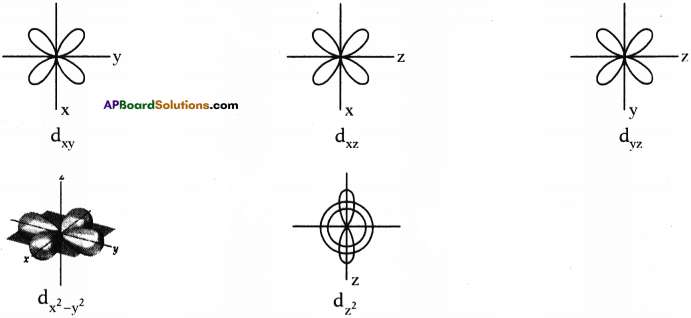Question 20.
Draw electromagnetic wave and label its parts.